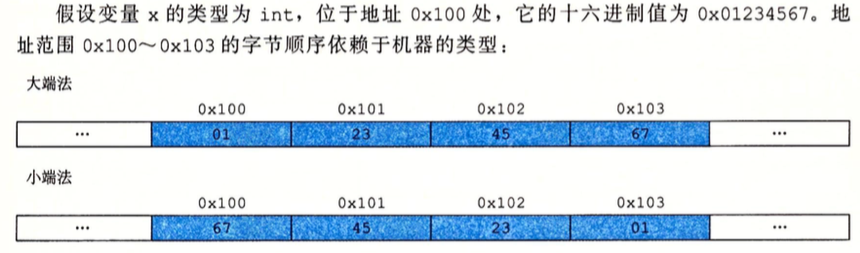# 2.1子数据大小

## 2.1.3 寻址和字节顺序

• 大端法，小端法## 2.1.6 布尔代数简介

• 位向量表示有限集合

a = 01101001 表示集合 {0, 3, 5, 6}

b = 01010101 表示集合 {0, 2, 4, 6}

a & b = 01000001 表示集合 {0, 6}

## 2.2.3 补码编码

 = -1 2^3 + 1 2^2 + 1 * 2^1 + 1 = -1

- =  -  = 

## 2.2.4 有符号和无符号之间的转换

unsigned u = 

## 2.2.6 拓展一个数字的位表示

• 无符号，左边加0
• 有符号，左边加最高有效位的值

# 2.3 整数运算

## 2.3.1 无符号加法

• 溢出
考虑一个4位数字 x =  y = , x + y =  丢弃最高位得到 

# 2.4 浮点数

## 2.4.2 IEEE浮点表示

V = (-1)^S M 2^e

s: 0 表示正数，1表示负数